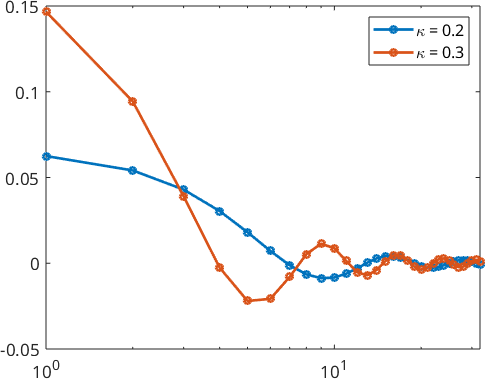Spherical Kernel Functions edit page

A spherical kernel $$\psi$$ is a spherical function that depends only on the angle towards the north pole $$e_3$$,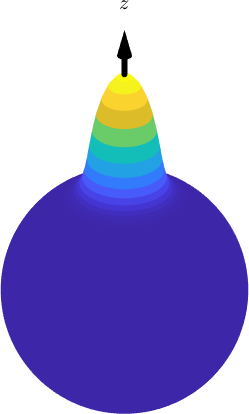The dependency of the angle becomes more when plot along meridian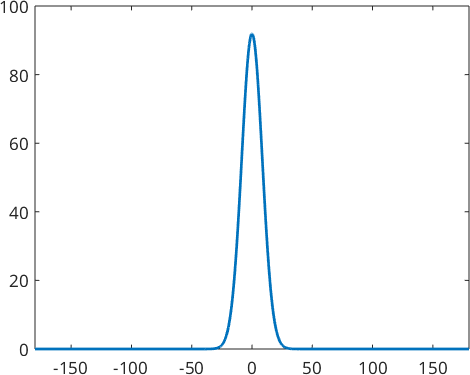Examples of spherical kernel functions are

• the de la Vallee Poussin kernel @S2DeLaValleePoussinKernel
• the Schulz defocusing kernel @SchulzDefocusingKernel
• the Dirichlet kernel @S2DirichletKernel
• the Bump kernel @S2BumpKernel
• the Abel Poussin kernel @S2AbelPoussinKernel.html de >
• the vom Mises kernel

## Legendre coefficients

Every spherical kernel function $$\psi$$ can be associated with a function $$\Psi \colon [-1,1] \to \mathbb R$$ defined on the interval $$[-1,1]$$ by $$\psi(v) = \Psi(v \cdot e_3)$$. It turn out to be usefull to $$\Psi$$ approximate $$\Psi$$ by a expansion into Legendre polynomials $$P_n$$ of degree $$n$$, i.e.,

$\psi(t) = \sum\limits_{n=0}^{\infty} \hat\psi_n \, \mathcal P_{n}(t)$

These Legendre coefficients are stored as the field psi.A and can be easily visualized using the command plotSpectra.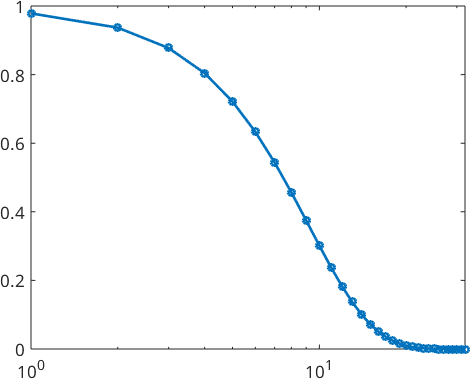## Applications

Spherical kernel functions have different applications in MTEX. Those include

## The de La Vallee Poussin Kernel

The spherical de la Vallee Poussin kernel is defined by

$K(t) = (1+\kappa)\,(\frac{1+t}{2})^{\kappa}$

for $$t\in[0,1]$$. The de la Vallee Poussin kernel additionaly has the unique property that for a given halfwidth it can be described exactly by a finite number of Fourier coefficients. This kernel is recommended for Texture analysis as it is always positive and there is no truncation error in Fourier space.

Hence we can define the de la Vallee Poussin kernel $$\psi_{\kappa}$$ depending on a parameter $$\kappa \in \mathbb N \setminus \{0\}$$ by its finite Legendre polynomial expansion

$\psi_{\kappa}(t) = \sum\limits_{n=0}^{L} a_n(\kappa) \mathcal P_{n}(t).$

We obtain the Legendre coefficients $$a_n(\kappa)$$ by $$a_0=1$$, $$a_1=\frac{\kappa}{2+\kappa}$$ and the three term recurence relation

$(\kappa+l+2) a_{l+1} = -(2l+1)\,a_l + (\kappa-l+1)\,a_{l-1}.$

Lets construct two of them.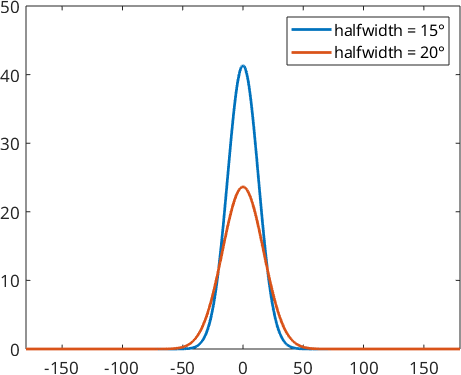Here the parameter $$\kappa$$ is $$40.34$$ for function $$\psi_1$$ and $$22.64$$ in function $$\psi_2$$.

We also take a look at the Legendre coefficients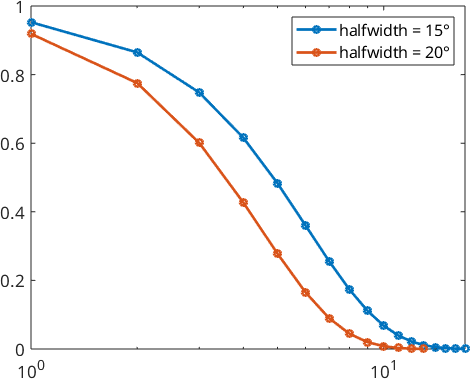## The Dirichlet Kernel

The spherical Dirichlet or Christoffel-Darboux kernel is recommended for calculating physical properties as the Legendre coefficients always have a value of one up to the specified bandwidth:

$\psi_N(t) = \sum\limits_{n=0}^N (2n+1) \, \mathcal P_{n}(t).$

Lets construct two of them.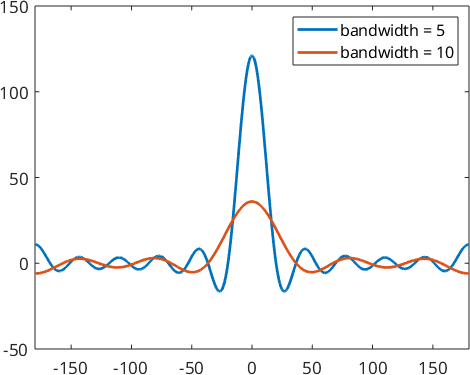By looking at the Legendre coefficients we see, that they are exactly 1.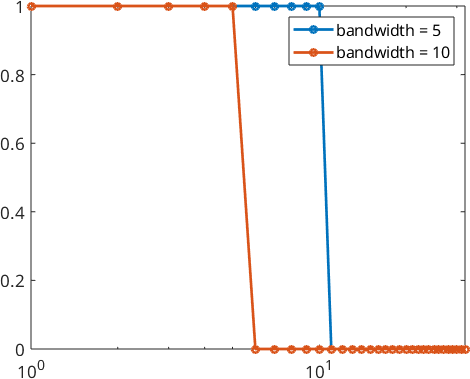## The Bump kernel

The spherical bump kernel is a radial symmetric kernel function depending on the halfwidth $$r\in (0,pi)$$. The function value is 0, if the angle is greater then the halfwidth $$r$$. Otherwise it is 1.

The main problem of the bump kernel is that we need lots of legendre coefficients to describe it. That possibly can result in high runtimes.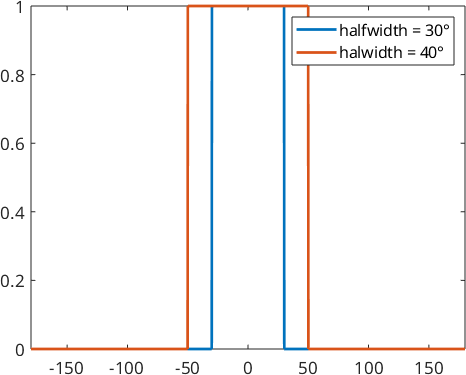We also take a look at the Fourier coefficients